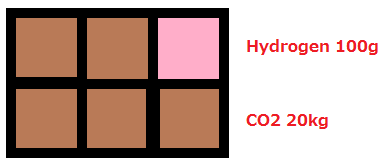## What do you think?   3 members have voted

1. ### 1. What do you think?

• Oh yeah!
0
• Maybe something like this can be done.
• Something can be done, but not this!
0
• It's fine as it is now, but could be better. Just not a priority.
0
• Don't touch that! Leave it the way it is.
0
• Dude, no... Go to sleep! :v

## Recommended Posts

Because only one gas can occupy a square at a time, it's not possible to measure the actual pressure of a room, unless it only have one gas.

For example:In this case, the hydrogen at the top keeps moving left/right. If I have a Atmo Sensor at the top, when the hydrogen is on top of it, it will detect only 100g, which is only true for that square. And depending on the automation it can cause some problems.

Maybe add a new measurement that averages the pressure within a room.
In this example is easy to do that, because it's very small.
5 squares of 20kg, 1 square of 100g, so... about 16,68kg per square.
This can be done in this example but I get it's not that easy in the game because the space is not that well enclosered and small.

What is (maybe) easier to be done is to average the pressure only of the neighbors, and only do so if the square has an Atmo Sensor (and maybe on the square below the mouse?), as it would be useless to calculate for everything all the time and that would only use precious cpu cycles to something that is not needed in those squares.

So, in the example:
Assuming I have a sensor in the hydrogen square.
What it would do is average the values of the hydrogen square with the neighbors squares (left, top, right, bottom).
In that case top and right are walls, so don't use them. So:
( 20kg (left) + 20kg (bottom) + 0,1kg (itself) ) / 3 = 13,36kg. It's not accurate (maybe use a bigger radius), but it's more accurate than reporting 100g.

If also calculated on the square that the mouse is over, this new measurement could be shown below the current one (and not replace it).

Just a though I had. Sorry if it's comepletely stupid.##### Share on other sites

Build 2 sensors and AND gate to solve your problem.

Calculation average values is waste of PC power because there is many different factors that have to be take into account.

3 hours ago, Alpe12 said:

So, in the example:
Assuming I have a sensor in the hydrogen square.
What it would do is average the values of the hydrogen square with the neighbors squares (left, top, right, bottom).
In that case top and right are walls, so don't use them. So:
( 20kg (left) + 20kg (bottom) + 0,1kg (itself) ) / 3 = 13,36kg. It's not accurate (maybe use a bigger radius), but it's more accurate than reporting 100g.

Right now calculation is easy, just measure pressure in one tile under sensor.

Check it top tile have pressure -> solid tile -> x1=0, y=y+1
Check if left tile have pressure -> CO2 -> x2=2000
Check if center tile have pressure -> H2 -> x3=100
Check if right tile have pressure -> solid tile -> x4=0, y=y+1
Check if bottom tile have pressure -> CO2 -> x5=2000
Calculate (x1+...x5)/(5-y)=4100/3=1337

See how much more calculations is needed for every sensor. The bigger radius the more calculations need to be done.